Ex 13.4

Chapter 13 Class 12 Probability
Serial order wise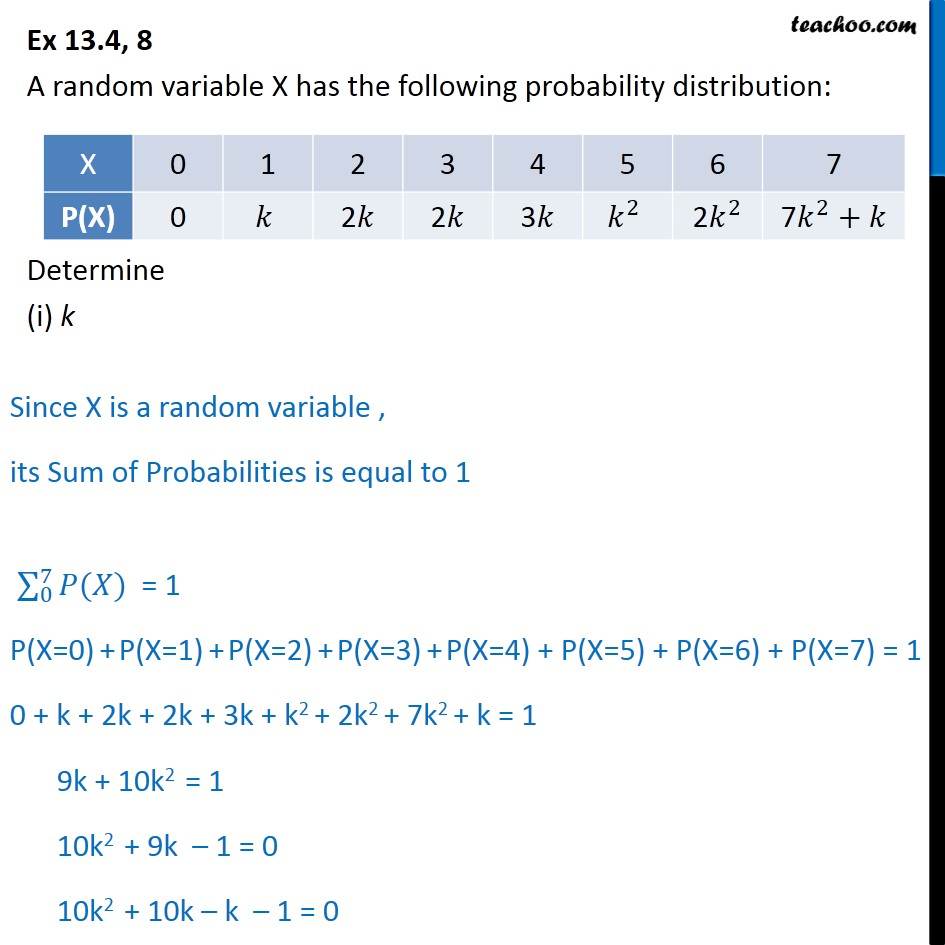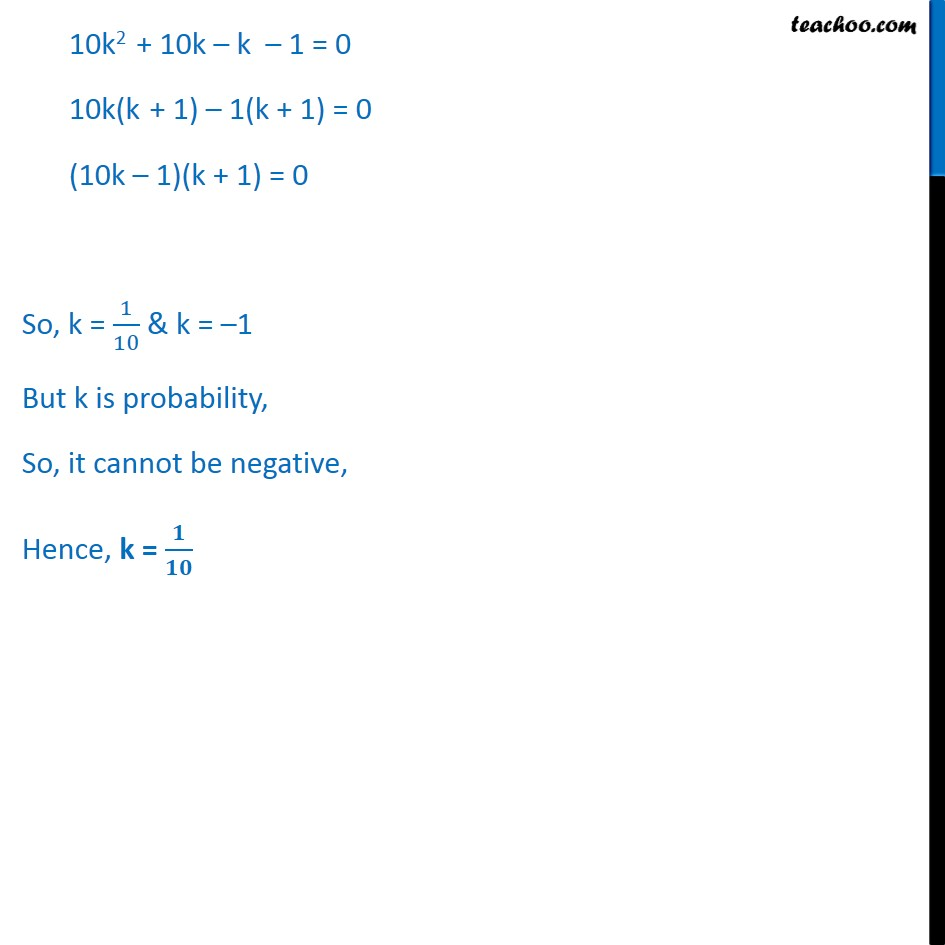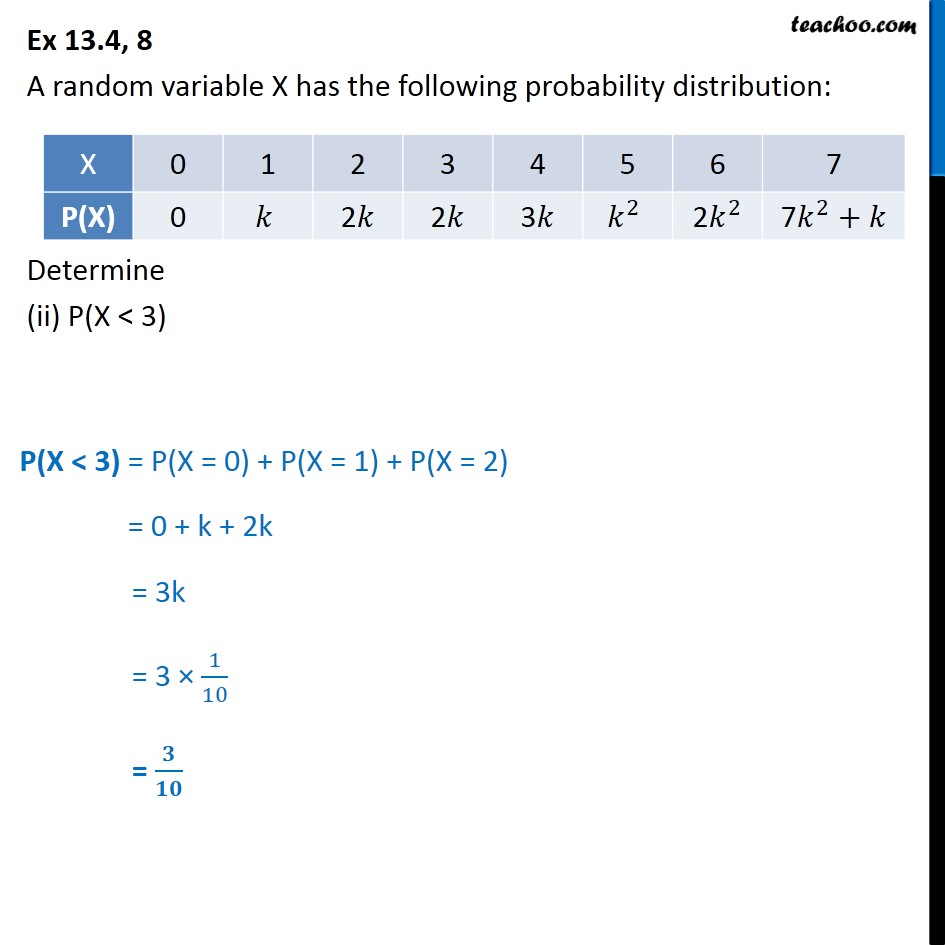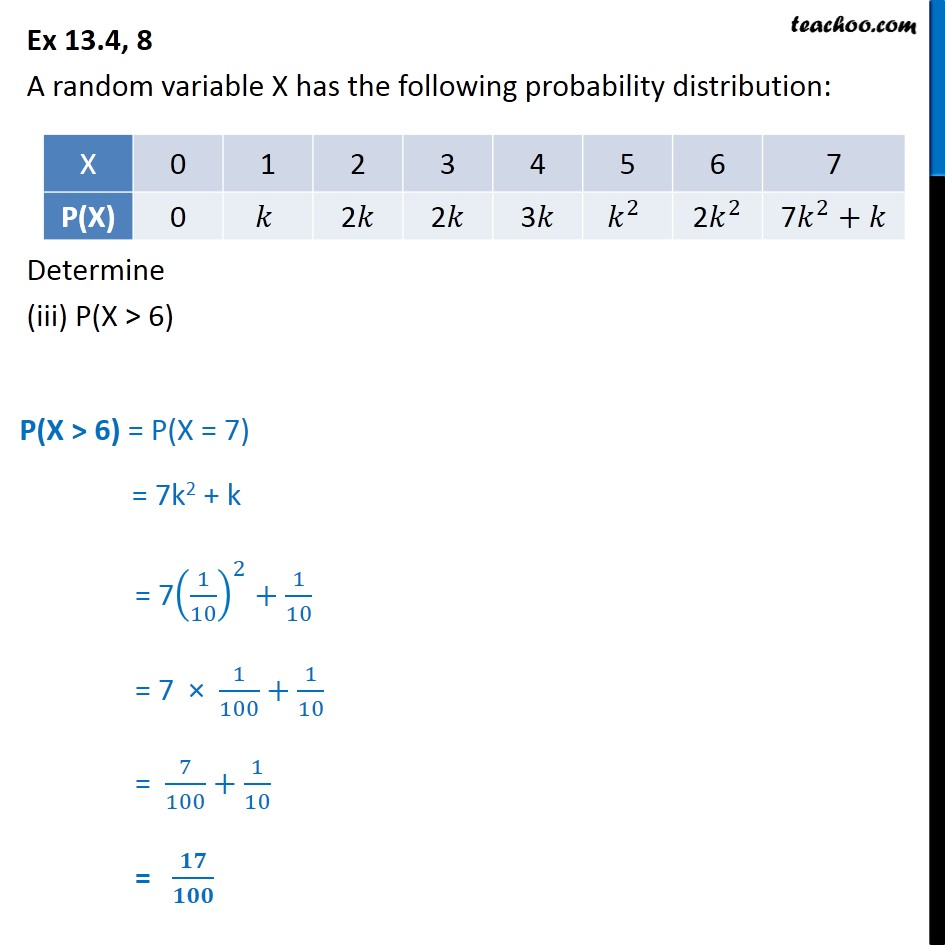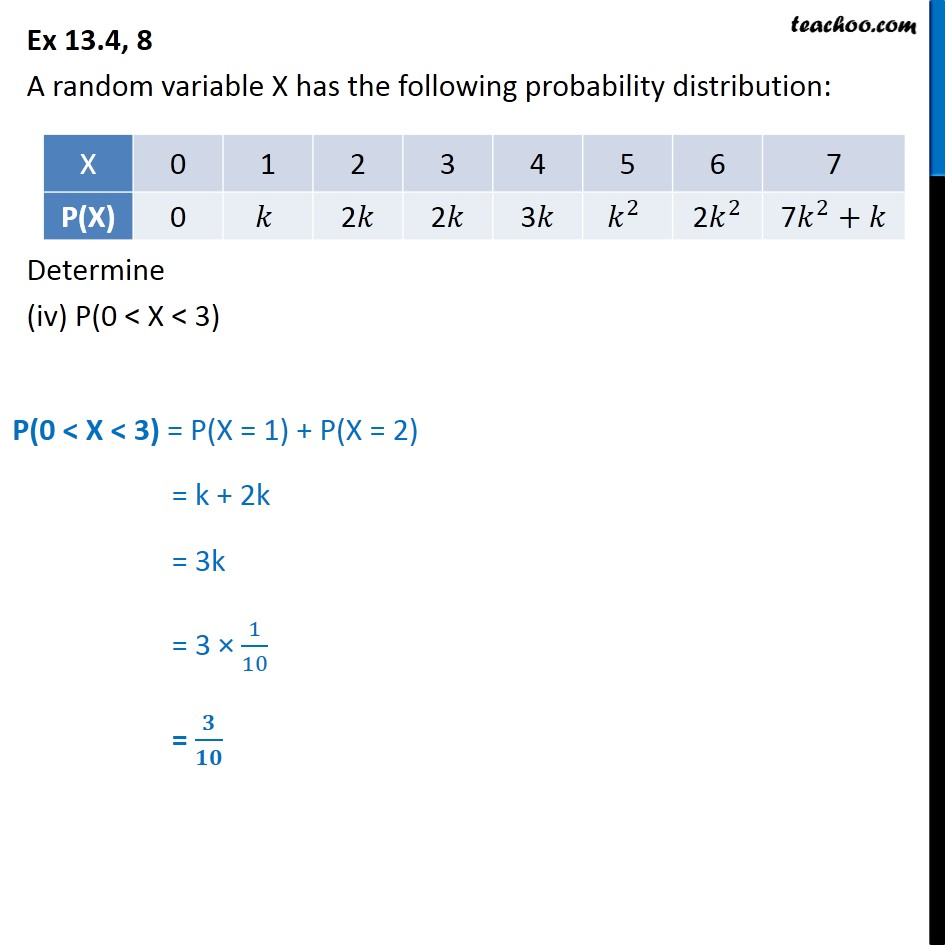Get live Maths 1-on-1 Classs - Class 6 to 12

### Transcript

Ex 13.4, 8 A random variable X has the following probability distribution: Determine (i) k Since X is a random variable , its Sum of Probabilities is equal to 1 0﷮7﷮𝑃(𝑋)﷯ = 1 P(X=0) + P(X=1) + P(X=2) + P(X=3) + P(X=4) + P(X=5) + P(X=6) + P(X=7) = 1 0 + k + 2k + 2k + 3k + k2 + 2k2 + 7k2 + k = 1 9k + 10k2 = 1 10k2 + 9k – 1 = 0 10k2 + 10k – k – 1 = 0 10k2 + 10k – k – 1 = 0 10k(k + 1) – 1(k + 1) = 0 (10k – 1)(k + 1) = 0 So, k = 1﷮10﷯ & k = –1 But k is probability, So, it cannot be negative, Hence, k = 𝟏﷮𝟏𝟎﷯ Ex 13.4, 8 A random variable X has the following probability distribution: Determine (ii) P(X < 3) P(X < 3) = P(X = 0) + P(X = 1) + P(X = 2) = 0 + k + 2k = 3k = 3 × 1﷮10﷯ = 𝟑﷮𝟏𝟎﷯ Ex 13.4, 8 A random variable X has the following probability distribution: Determine (iii) P(X > 6) P(X > 6) = P(X = 7) = 7k2 + k = 7 1﷮10﷯﷯﷮2﷯+ 1﷮10﷯ = 7 × 1﷮100﷯+ 1﷮10﷯ = 7﷮100﷯+ 1﷮10﷯ = 𝟏𝟕﷮𝟏𝟎𝟎﷯ Ex 13.4, 8 A random variable X has the following probability distribution: Determine (iv) P(0 < X < 3) P(0 < X < 3) = P(X = 1) + P(X = 2) = k + 2k = 3k = 3 × 1﷮10﷯ = 𝟑﷮𝟏𝟎﷯